Examples from NCERT Book

Class 10
Chapter 10 Class 10 - Light - Reflection and Refraction

## A 2.0 cm tall object is placed perpendicular to the principal axis of a convex lens of focal length 10 cm. The distance of the object from the lens is 15 cm. Find the nature, position and size of the image. Also find its magnification.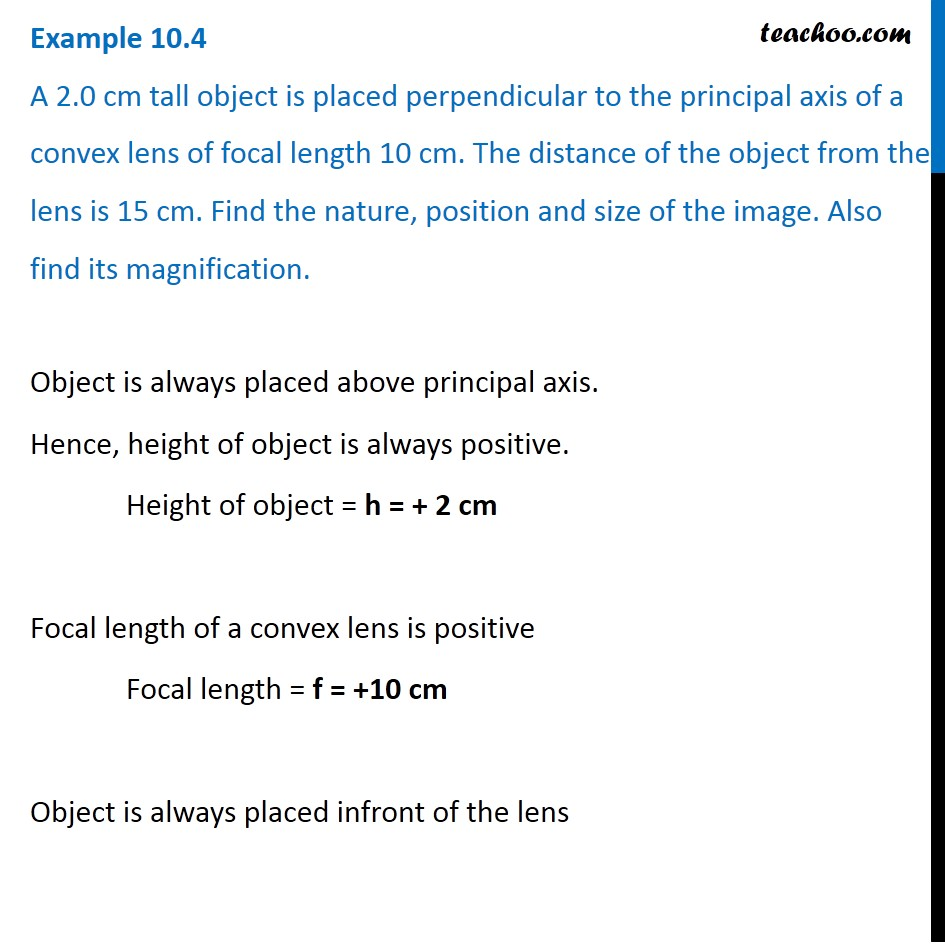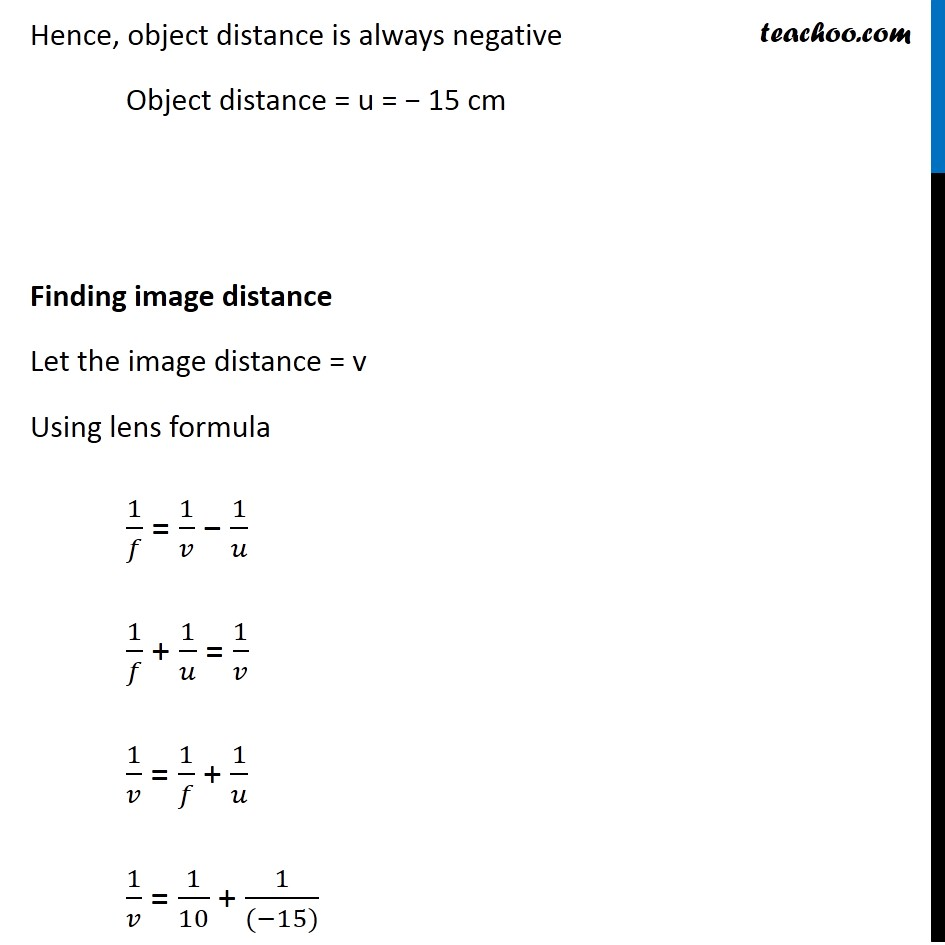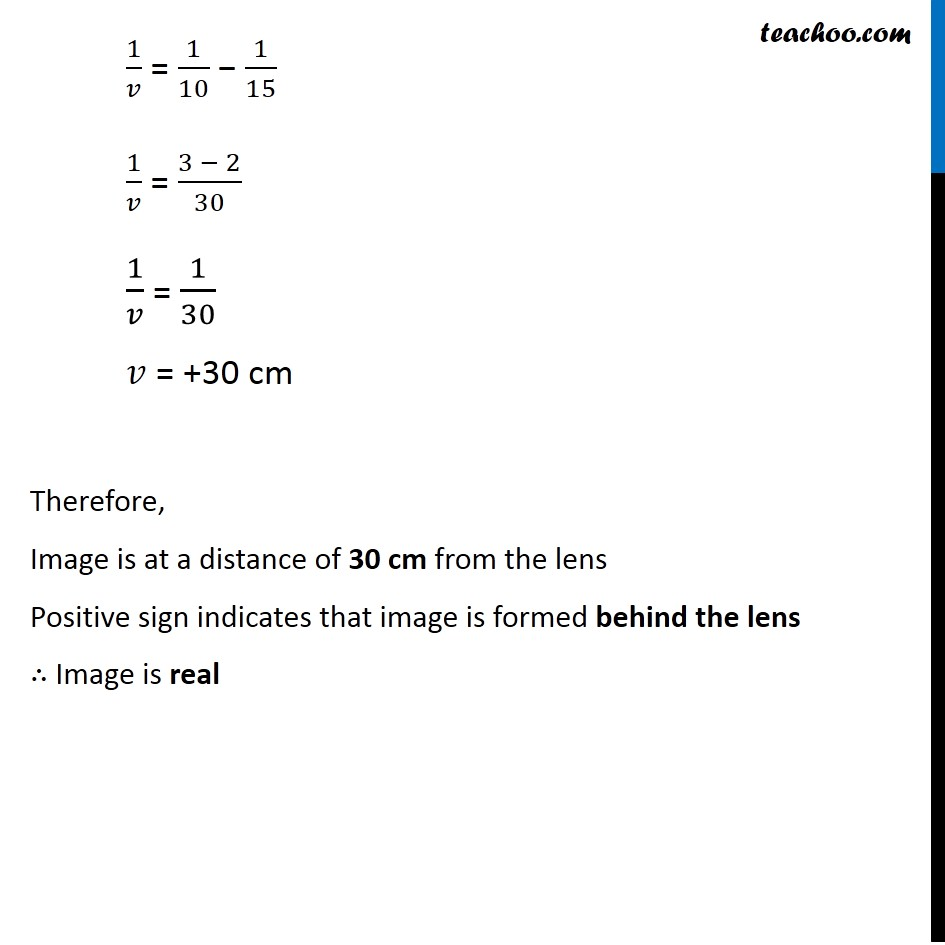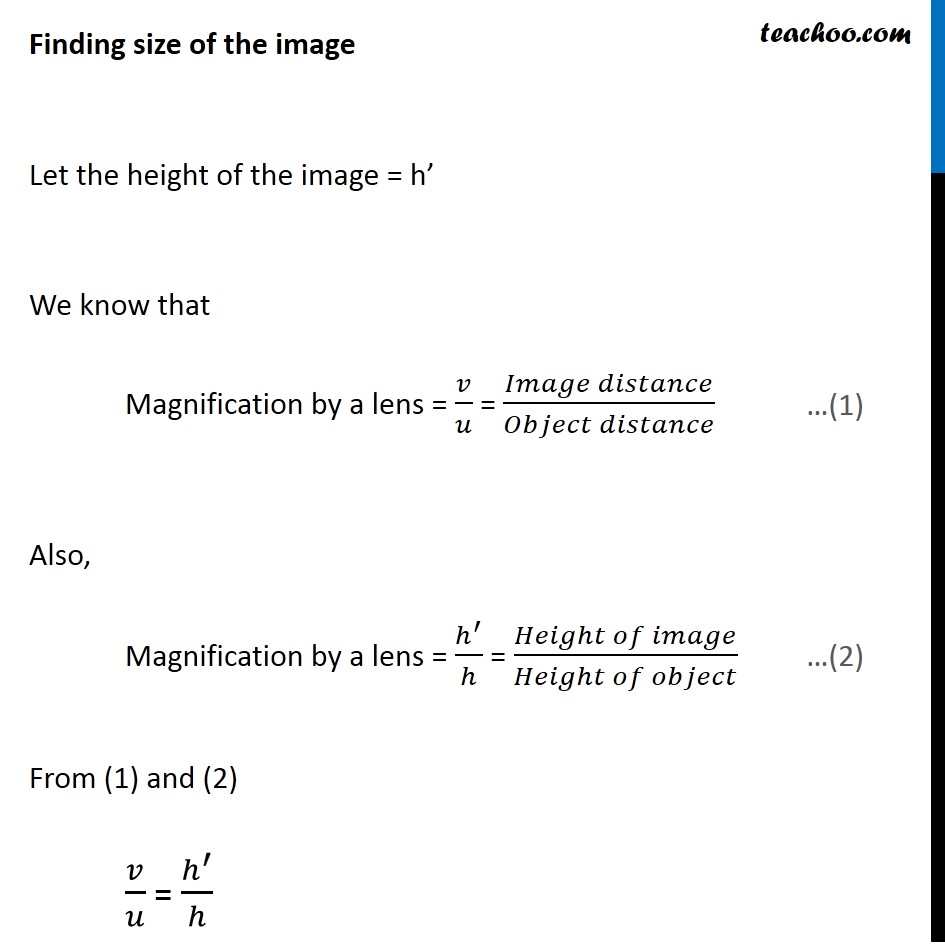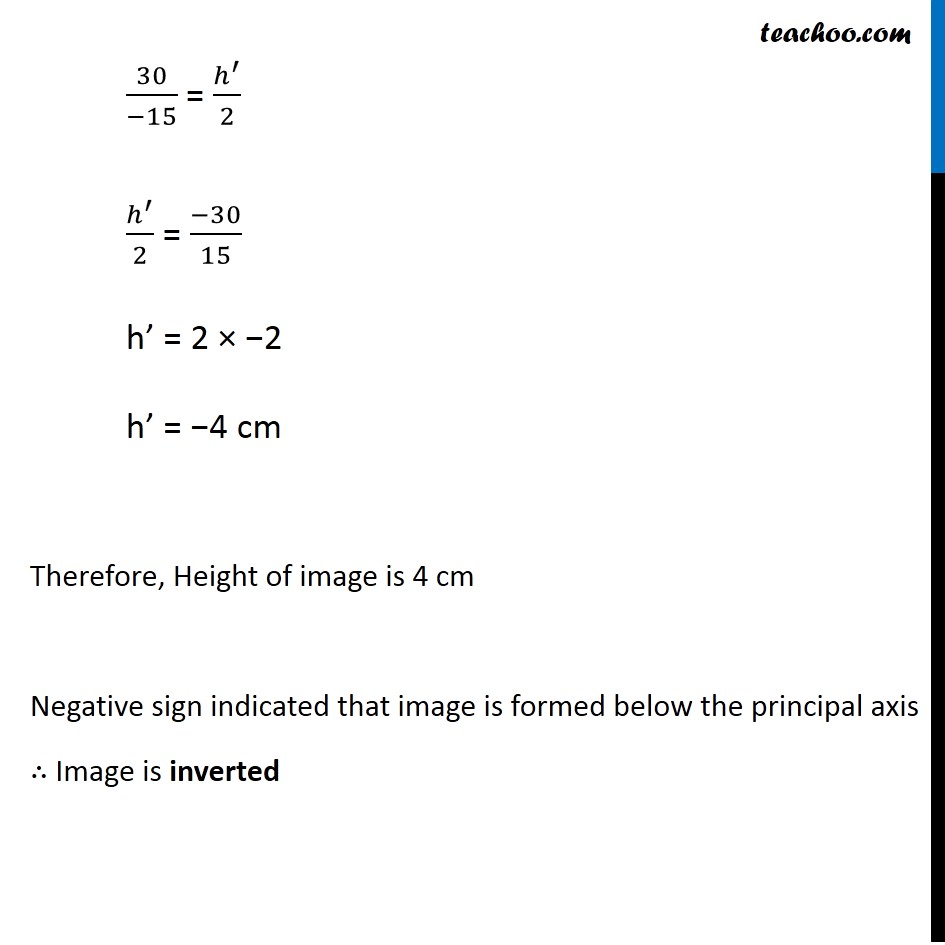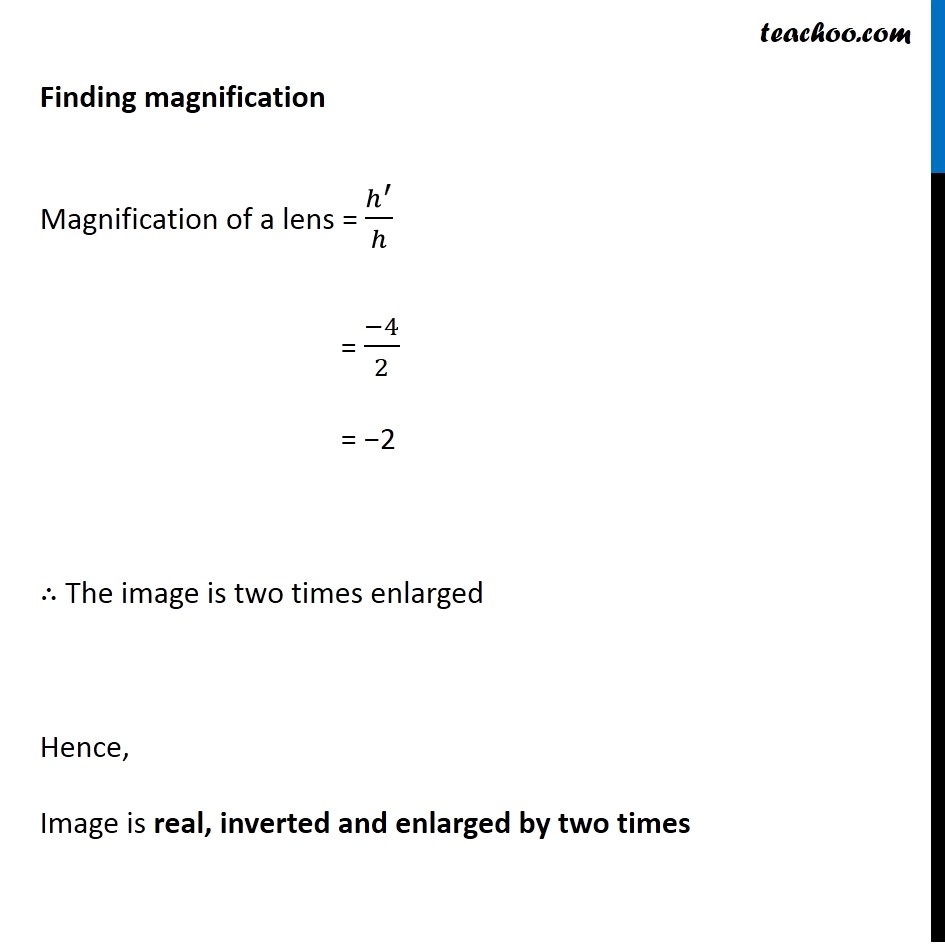Learn in your speed, with individual attention - Teachoo Maths 1-on-1 Class

### Transcript

A 2.0 cm tall object is placed perpendicular to the principal axis of a convex lens of focal length 10 cm. The distance of the object from the lens is 15 cm. Find the nature, position and size of the image. Also find its magnification. Object is always placed above principal axis. Hence, height of object is always positive. Height of object = h = + 2 cm Focal length of a convex lens is positive Focal length = f = +10 cm Object is always placed infront of the lens Hence, object distance is always negative Object distance = u = − 15 cm Finding image distance Let the image distance = v Using lens formula 1/𝑓 = 1/𝑣 − 1/𝑢 1/𝑓 + 1/𝑢 = 1/𝑣 1/𝑣 = 1/𝑓 + 1/𝑢 1/𝑣 = 1/10 + 1/((−15)) 1/𝑣 = 1/10 − 1/15 1/𝑣 = (3 − 2)/30 1/𝑣 = 1/30 𝑣 = +30 cm Therefore, Image is at a distance of 30 cm from the lens Positive sign indicates that image is formed behind the lens ∴ Image is real Finding size of the image Let the height of the image = h’ We know that Magnification by a lens = 𝑣/𝑢 = (𝐼𝑚𝑎𝑔𝑒 𝑑𝑖𝑠𝑡𝑎𝑛𝑐𝑒)/(𝑂𝑏𝑗𝑒𝑐𝑡 𝑑𝑖𝑠𝑡𝑎𝑛𝑐𝑒) Also, Magnification by a lens = ℎ^′/ℎ = (𝐻𝑒𝑖𝑔ℎ𝑡 𝑜𝑓 𝑖𝑚𝑎𝑔𝑒)/(𝐻𝑒𝑖𝑔ℎ𝑡 𝑜𝑓 𝑜𝑏𝑗𝑒𝑐𝑡) From (1) and (2) 𝑣/𝑢 = ℎ^′/ℎ 30/(−15) = ℎ^′/2 ℎ^′/2 = (−30)/15 h’ = 2 × −2 h’ = −4 cm Therefore, Height of image is 4 cm Negative sign indicated that image is formed below the principal axis ∴ Image is inverted Finding magnification Magnification of a lens = ℎ^′/ℎ = (−4)/2 = −2 ∴ The image is two times enlarged Hence, Image is real, inverted and enlarged by two times